Date: Tue, 14 Oct 1997 18:19:29 -0400
Sender: David
Subject: Middle School Level Math Question (HELP!!)

I am a teacher in Massachusettes. We have been diagramming numbers such as two to the third (a 3-D cube). One of my students asked me how you would diagram two to the fourth. I have searched through all of my teachers books and cannot seem to find the answer to this question. Is there a way to diagram this? If there is, how would you do this?

Your help would be greatly appreciated.

Thank you,

David

Hi David,

I would use a tree diagram to illustrate powers. For example for powers of 2 start with a tree with two branches.To diagram 2^2=2*2 double the number of paths through the tree by adding a second level of branches to the tree.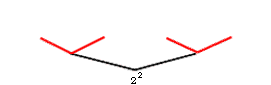For 2^3=2(2^2) again double the number of paths through the tree by adding a thrid level.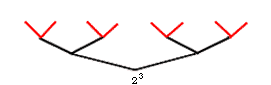And for 2^4=2(2^3).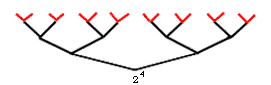Conceptually you can continue this process but the tree soon becomes unmanagable.

Powers with a base other than 2 can also be described with a tree diagram, for example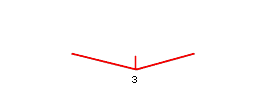and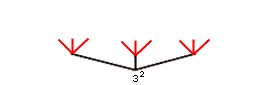Trees can be useful in many areas of mathematics. For example the tree for 2^3 can be used to describe all possibilities when a coin is tossed three times.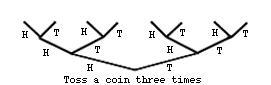Cheers,
Penny

Go to Math Central

To return to the previous page use your browser's back button.